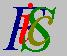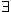Mauro Murzi's pages on Philosophy of Science - Logical Positivism
Synthetic and Analytic Statements

# [2. The Main Philosophical Tenets of Logical Positivism.]

## e. Synthetic and Analytic Statements.

The solution to the problem about the meaning of theoretical terms that Carnap proposed in "Beobacthungssprache und theoretische Sprache" in Dialectica, 12, 1958 (English translation "Observation language and theoretical language" in Rudolf Carnap, Logical Empiricist, 1975) was based on the analytic-synthetic distinction. Carnap's method explains the meaning of theoretical terms and their relationships with observational concepts, gives a method for separating synthetic and analytic sentences, and gives a method for dividing theoretical axioms from rules of correspondence.

Suppose the number of theoretical axioms is finite. Let T be their conjunction and let C the conjunction of all rules of correspondance; let TC the conjunction of T and C. The theory is equivalent to the single axiom TC. Carnap formulates the following problem: how can we find two statements, say A and R, so that A expresses the analytic portion of the theory (i.e., all consequences of A are analytic) while R expresses the empirical portion (i.e., all consequences of R are synthetic)? The empirical content of the theory is formulated by means of a Ramsey sentence (a discovery of the English philosopher Frank Ramsey). Carnap's solution to the problem builds a Ramsey sentence on the following instructions:

1. Replace every theoretical term in TC with a variable.
2. Add an appropriate number of existential quantifiers at the beginning of the sentence.

Look at the following example. Let TC(O1 ... On , T1 ... Tm) be the conjunction of T and C; in TC there are observational terms O1 ... On and theoretical terms T1 ... Tm. The Ramsey sentence (R) isX1 ...Xm TC(O1 ... On , X1 ... Xm)

Every observational statement which is derivable from TC is also derivable from R and vice versa; thus R expresses exactly the empirical portion of the theory. Carnap proposes the statement R → TC as the only rules of correspondence; this became known as the Carnap sentence, and it is the searched sentence A expressing the analytic portion of the theory. Note that every empirical statement that can be derived from the Carnap sentence is logically true, and thus the Carnap sentence lacks empirical consequences. So, a statement is analytic if it is derivable from the Carnap sentence; otherwise the statement is synthetic. The requirements of Carnap's method can be summarized as follows: (i) non-logical axioms must be explicitly stated, (ii) the number of non-logical axioms must be finite and (iii) observational terms must be clearly distinguished from theoretical terms.